• +91 9971497814
• info@interviewmaterial.com

# RD Chapter 21- Surface Areas and Volume of a Sphere Ex-21.1 Interview Questions Answers

### Related Subjects

Question 1 :
Find the surface area of a sphere of radius.
(i) 10.5 cm
(ii) 5.6 cm
(iii) 14 cm

Answer 1 : In a sphere,
(i) Radius (r) = 10.5 cm
Surface area = 4πr2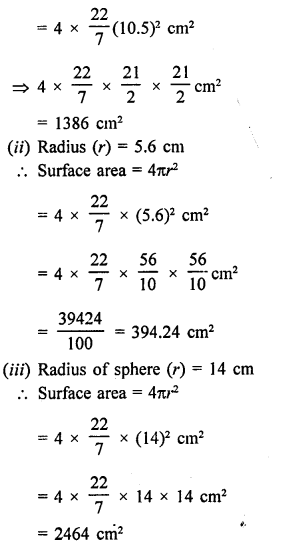Question 2 :
Find the surface area of a sphere of diameter
(i) 14 cm
(ii) 21 cm
(iii) 3.5 cm

(i) Diameter of a sphere = 14 cm
Radius (r) = 14/2 = 7 cm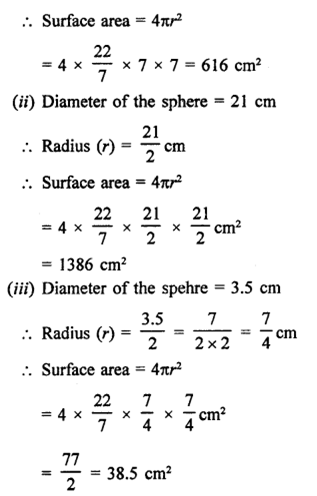Question 3 : Find the total surface area of a hemisphere and a solid hemisphere each of radius 10 cm. [Use π = 3.14]

(i) Radius of hemisphere = 10 cm
Totalsurface area of hemisphere = 2πr2
= 2 x 3.14 x 10 x 10 cm2
= 628 cm2
(ii) Total surface area of solid hemisphere
= 3πr2 = 3 x 3.14 x 10 x 10 cm2
= 942 cm2

Question 4 : The surface area of a sphere in 5544 cm2, find the diameter.

Answer 4 : Let r be the radius of a sphere, then Surface area =4πr2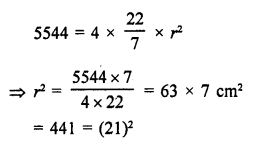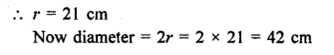Question 5 : A hemispherical bowl made of brass has inner diameter 10.5 cm. Find the cost of tin-plating it on the inside at the rate of ₹4 per 100 cm2. [NCERT]

Answer 5 : Inner diameter of a hemispherical bowl = 10.5 cm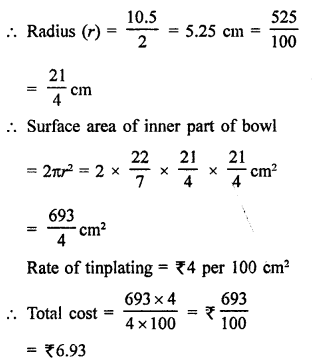Question 6 : The dome of a building is in the form of a hemisphere. Its radius is 63 dm. Find the cost of painting it at the rate of ₹2 per sq. m.

Radius of dome (hemispherical) = 63 dm
Area of curved surface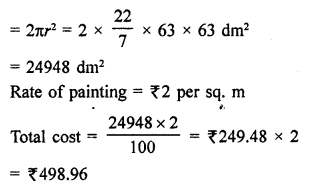Question 7 : Assuming the earth to be a sphere of radius 6370 km, how many square kilometres is area of the land, if three-fourth of the earth’s surface is covered by water?

Radius of earth (sphere) = 6370 km
Water on the earth = 3/4 % total area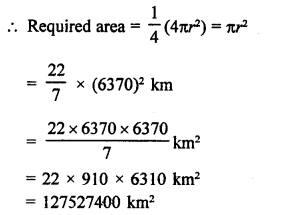Question 8 : A cylinder of same height and radius is placed on the top of a hemisphere. Find the curved surface area of the shape if the length of the shape be 7 cm.

Answer 8 : Total height of the so formed shape = 7 cm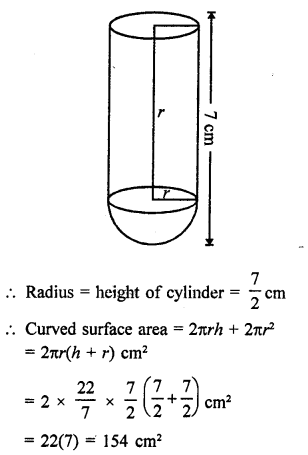Question 9 : The diameter of the moon is approximately one fourth of the diameter of the earth. Find the ratio of their surface areas.

Answer 9 : Diameter of moon = 1/4 of diameter of earth
Let radius of earth = r km
1/4 r km
Now surface area of earth = 4πr2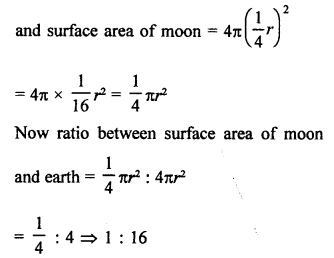Question 10 : A hemi-spherical dome of a building needs to be painted. If the circumference of the base of the dome is 17.6 m, find the cost of painting it, given the cost of painting is ₹5 per 100 cm2. [NCERT]

Answer 10 : Circumference of the base of dome (r) = 17.6 m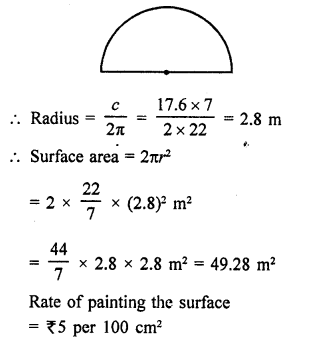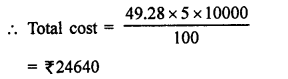Todays Deals### RD Chapter 21- Surface Areas and Volume of a Sphere Ex-21.1 Contributorskrishan

Name:
Email:

# Latest News# 9000 interview questions in different categories